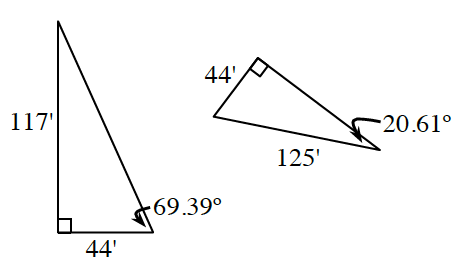### Home > GB8I > Chapter 7 Unit 8 > Lesson INT1: 7.1.1 > Problem7-7

7-7.

Are the two triangles below congruent? Make a sketch of both triangles, then determine all of the angle measures and all of the side lengths. If they are congruent, describe a sequence of rigid transformations that maps one onto the other. Note: The triangles are not drawn to scale.Use the Pythagorean Theorem to determine the lengths of any unknown sides.

Remember that all the internal angles of a triangle add to $180º$.
Try creating an equation to determine the measures of the unknown angles.

Now that you know the unknowns, are the triangles congruent?